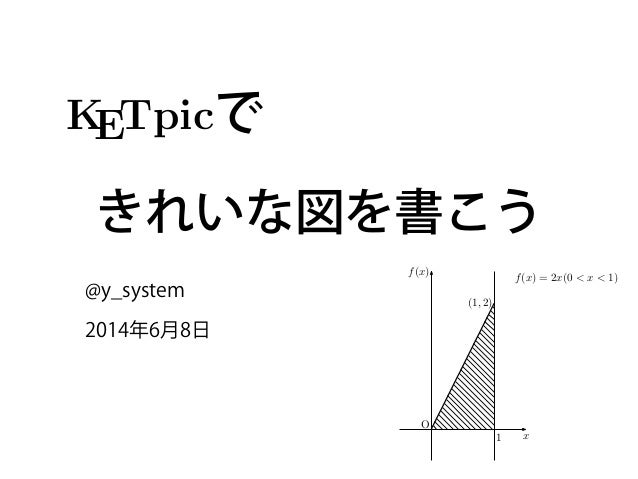Successfully reported this slideshow.Upcoming SlideShare
×

# KETpic できれいな図を書こう

2,143 views

Published on

KETpic を使って TeX に教科書のようなきれいな図を挿入しようというお話です．
KETpic では3次元のものだとか細かい機能が定義されていますが，簡単な作図を用いてこのスライドでは解説していきます．

Published in: Design
• Full Name
Comment goes here.

Are you sure you want to Yes No### KETpic できれいな図を書こう

1. 1.    で きれいな図を書こう @y_system 2014年6月8日 KETpic v4.2.2 ltd コマンド一 for R いての注意 込みには次を実行する． /work/ketpic.Rdata”)  （C:/work/は作業フォルダ名） ィレクトリの変更は setwd(”c:/work”) (1, 2) f(x) = 2x(0 < x < 1) 1 x f(x) O
2. 2. このスライドは 統計解析ソフトR で     を使って，TEXの作図を 美しくしようというもの どんなに頑張ってもRのplotでは限界がある！ Rではexpression関数で数式表現はサポートされてま すが…見た目は美しくない… 2 2 KETpic v4.2.2 ltd コマンド一覧 for R PD 1 R についての注意 1. KETpic の読込みには次を実行する． load(”C:/work/ketpic.Rdata”)  （C:/work/は作業フォルダ名） ※ ディレクトリの変更は setwd(”c:/work”) 2. 文字列は  ”（ダブル）で囲む ※ 文字列の中に文字列を入れるときは ’ と ” を入れ子に使う． 3. 関数などを引数とするときは，文字列とする． 例）G<- Plotdata( ”x^2”, ”x=c(0,1)”) 4. （バックスラッシュ）を出力するには２つ並べてかく． 5. 注釈は # 6. 数と文字列の変換 as.character(数) f(x)=x 2
3. 3. about: 教科書のようなきれいな図をTEXに 図の中の記号や数式も本文と同じ見た目 図をわかりやすく！ 3 KETpic v4.2.2 ltd コマン for R R についての注意 KETpic の読込みには次を実行する． load(”C:/work/ketpic.Rdata”)  （C:/work/は作業フォルダ名） ※ ディレクトリの変更は setwd(”c:/work”) 文字列は  ”（ダブル）で囲む ※ 文字列の中に文字列を入れるときは ’ (1, 2) f(x) = 2x(0 < x < 1) 1 x f(x) O
4. 4. about: TEXには数値計算の十分な機能はないので解析ソフト などで計算を行う Rの上でKETpic関数を利用してTEX用の作図を得ると いうもの 4 KETpic v4.2.2 ltd コマン for R R についての注意 KETpic の読込みには次を実行する． load(”C:/work/ketpic.Rdata”)  （C:/work/は作業フォルダ名） ※ ディレクトリの変更は setwd(”c:/work”) 文字列は  ”（ダブル）で囲む ※ 文字列の中に文字列を入れるときは ’ R TeX 図ファイル .texの作成 KETpic 関数 KETpic スタイルファイル (1, 2) f(x) = 2x(0 < x < 1) 1 x f(x) O 1 f(x) = 2x(0 < x < 1) f(x) = x2 (0 < x < 1) f(x)
5. 5. 準備 TeXとRは既にインストールしてあると思うので， 必要なのは， • Rの関数が定義されたファイル • TeXのスタイルファイル このファイルがないと始まらない！ 5
6. 6. まず，公式サイトに行きましょう まず http://ketpic.com/ にアクセスし， ダウンロードをクリック 6
11. 11. それぞれ解凍しましょう さきほどダウンロードしたファイルをダブルクリック などで解凍してあげましょう 11
12. 12. 解凍したら… こんなファイル構成にしましょう． 今回はstyのインストールはしません． 12 どこかのフォルダ あなたのtexファイル ketpic.sty ketpicフォルダ ketpic_ltd.Rdata 作成した図ファイル
13. 13. 作図データをつくろう ketpic_ltd.Rdata をダブルクリックしてRを 起動します． （既に起動済みの方はDrag&Dropなどで） 13
14. 14. 作図データをつくろう このコードをRで実行してみよう 14 Ketinit() Setwindow(c(-0.5,1.5),c(-0.5,2.5)) G=Plotdata('2*x','x=c(0,1)') Gv=Listplot(c(1,0),c(1,2)) SEG1=Listplot(c(0,0),c(1,0),c(1,2)) Windisp(G) Openfile("2x.tex") Beginpicture('20mm') Drwline(G,2) Drwline(Gv,0.5) Setax("a0.5","x","s2","f(x)","w","O","nw") Expr(c(1,2),"w","(1,2)") Expr(c(1.3,2.4),"e","f(x)=2x (0<x<1)") Expr(c(1,0),"es2","1") GH=Hatchdata(list('ii'),list(SEG1,'n'),list(G,'s'),-45,0.5) Drwline(GH,0.5) Endpicture(1) Closefile()
15. 15. 作図データをつくろう このコードをRで実行してみよう Ketinit() Setwindow(c(-0.5,1.5),c(-0.5,2.5)) G=Plotdata('2*x','x=c(0,1)') Gv=Listplot(c(1,0),c(1,2)) SEG1=Listplot(c(0,0),c(1,0),c(1,2)) Windisp(G) Openfile("2x.tex") Beginpicture('20mm') Drwline(G,2) Drwline(Gv,0.5) Setax("a0.5","x","s2","f(x)","w","O","nw") Expr(c(1,2),"w","(1,2)") Expr(c(1.3,2.4),"e","f(x)=2x (0<x<1)") Expr(c(1,0),"es2","1") GH=Hatchdata(list('ii'),list(SEG1,'n'),list(G,'s'),-45,0.5) Drwline(GH,0.5) Endpicture(1) Closefile() 15 データの 作成 作図TeX 書き出し 細かいコードの説明は後で
16. 16. TeX に図を挿入しよう 先ほど「2x.tex」が作成されたと思うので， 本文となるtexファイルをketpic.styと同じフォルダに つくりましょう． 16
17. 17. TeX のソース ketpic と graphicx は必須！ ﬁgure 環境の中にinputコマンドで先ほどの作図を入 れる． 17 documentclass[12pt]{jsarticle} usepackage{ketpic} usepackage{graphicx} usepackage{float} begin{document} begin{figure}[H] centering input{ketpic/2x.tex} caption{\$f(x)=2x (0<x<1)\$のグラフ} end{figure} end{document}
18. 18. コンパイル こんなPDFが出力されたと思います！ ! ! ! ! ! ! ! 次のページで解説 18 (1, 2) f(x) = 2x(0 < x < 1) 1 x f(x) O 図 1 f(x) = 2x(0 < x < 1) のグラフ 1
19. 19. コードの解説 Ketinit() Setwindow(c(-0.5,1.5),c(-0.5,2.5)) G=Plotdata('2*x','x=c(0,1)') Gv=Listplot(c(1,0),c(1,2)) SEG1=Listplot(c(0,0),c(1,0),c(1,2)) Windisp(G) Openfile("2x.tex") Beginpicture('20mm') Drwline(G,2) Drwline(Gv,0.5) Setax("a0.5","x","s2","f(x)","w","O","nw") Expr(c(1,2),"w","(1,2)") Expr(c(1.3,2.4),"e","f(x)=2x (0<x<1)") Expr(c(1,0),"es2","1") GH=Hatchdata(list('ii'),list(SEG1,'n'),list(G,'s'),-45,0.5) Drwline(GH,0.5) Endpicture(1) Closefile() 19 データの 作成 作図TeX 書き出し
20. 20. Code for generating values 20 # 領域の初期化 Ketinit() # 作図領域の指定 Setwindow(c(-0.5,1.5),c(-0.5,2.5)) # 関数系のデータ作成 G=Plotdata(‘2*x','x=c(0,1)') # 線分データの作成 Gv=Listplot(c(1,0),c(1,2)) # 線分データの作成(頂点３つ以上で領域の作成) SEG1=Listplot(c(0,0),c(1,0),c(1,2)) データの 作成 ここまでがデータ座標データなどの準備
21. 21. Code for extracting the TeX source 21 # 書き出すファイルの指定 Openfile(“2x.tex") # 拡大率の設定（図の1が何mmなのか） Beginpicture(‘20mm') # 先ほど設定した線の書き出し Drwline(G,2) # y=2x Drwline(Gv,0.5) # 線分 # 軸関連の設定 # Setax(軸の形状,横軸名,横軸名の位置,縦軸名,縦軸名の位置, # 原点名,原点の位置) # a0.5 とすると線幅を指定できる Setax(“a0.5”,"x","s2","f(x)","w","O","nw") # 座標に文字を書き入れる # Expr(座標, 東西南北, TeXの数式表現) Expr(c(1,2),"w","(1,2)") Expr(c(1.3,2.4),"e","f(x)=2x (0<x<1)") Expr(c(1,0),”es2","1") # es2とすると右下の下 作図TeX 書き出し 続く 1/2
22. 22. Code for extracting the TeX source 22 # 領域の図示 # Hatchdata( 領域の内外,領域の指定:閉曲線や曲線の上下の指定, # 斜線の向き,線幅 ) GH=Hatchdata(list(‘ii'),list(SEG1,'n'),list(G,'s'),-45,0.5) # 描画 Drwline(GH,0.5) # 作図終了の宣言 # 1で座標軸を書く，0で書かずに終了． Endpicture(1) # Rでのファイル書き出しの終了の宣言． Closefile() 作図TeX 書き出し 2/2
23. 23. 他にも色々できます ketpicR_4_3_1_ltd01.zip の中の referenceR4_3_1_ltd.pdf に様々な関数の説明が書いてあります． 23 2014 年 1 月 2 日 KETpic v4.3.1 ltd コマンド一覧 for R PD プロットデータ 1 R についての注意 1. KETpic の読込みには次を実行する． load(”C:/work/ketpic.Rdata”)  （C:/work/は作業フォルダ名） ※ ディレクトリの変更は setwd(”c:/work”) 2. 文字列は  ”（ダブル）で囲む ※ 文字列の中に文字列を入れるときは ’ と ” を入れ子に使う． 3. 関数などを引数とするときは，文字列とする． 例）G<- Plotdata( ”x^2”, ”x=c(0,1)”) 4. （バックスラッシュ）を出力するには２つ並べてかく． 5. 注釈は # 6. 数と文字列の変換 as.character(数) as.numeric(文字列) eval(parse(text=文字列))) sprintf(書式, 数) 書式付き文字列 7. 異なる型のデータからリストを作るには list を用いる． 作成    L<- list(... , ... ); 取り出し  A<- L[[i]] 部分リスト  L[V] (V はベクトル) 置き換え  L[[i]]<- ... 長さ    length(L)
24. 24. 作成例
25. 25. 三乗根 Ketinit() Setwindow(c(-1.5,1.5),c(-1.5,1.5)) x=seq(0,2*pi,.01) G=matrix(c(cos(x),sin(x)),length(x),2) ! Windisp(G) Openfile("omega.tex") Beginpicture('2cm') Dashline(G,1) ! Setax("a0.7","rm Re","s0.7","rm Im","w","O","sw") Expr(c(1,0),"es2","1") Expr(c(cos(2*pi/3),sin(2*pi/3)),"wn","-displaystylefrac{1}{2}+frac{sqrt{3}}{2}i") Expr(c(cos(4*pi/3),sin(4*pi/3)),"ws","-displaystylefrac{1}{2}-frac{sqrt{3}}{2}i") Drwxy() Setpt(5) Drwpt(c(1,0),1) Drwpt(c(cos(2*pi/3),sin(2*pi/3)),1) Drwpt(c(cos(4*pi/3),sin(4*pi/3)),1) Endpicture(0) Closefile() 1 − 1 2 + √ 3 2 i − 1 2 − √ 3 2 i Re Im O 1
26. 26. Bibliography CASTEX応用研究会(2011), 『KETpicで楽々TEX グラフ』, イーテキスト研究所. Y. Maeda & M. Kaneko (2013). “Making math textbooks and materials with TEX+KETpic +hyperlink”, The 34th Annual Meeting of the TeX Users Group. 26